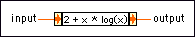# Expression Node

## LabVIEW 2018 Help

Edition Date: March 2018
Part Number: 371361R-01
View Product InfoLabVIEW 2016 HelpLabVIEW 2017 HelpLabVIEW 2018 HelpLabVIEW 2019 HelpLabVIEW 2020 Help

Owning Palette: Numeric Functions

Requires: Base Development System

Use the Expression Node to calculate expressions that contain a single variable. The following built-in functions are allowed in formulas: abs, acos, acosh, asin, asinh, atan, atanh, ceil, cos, cosh, cot, csc, exp, expm1, floor, getexp, getman, int, intrz, ln, lnp1, log, log2, max, min, mod, rand, rem, sec, sign, sin, sinc, sinh, sizeOfDim, sqrt, tan, tanh.input is the value that the Expression Node uses as the variable.output returns the value of the calculation.

## Expression Node Details

Expression Nodes are useful when an expression has only one variable but is otherwise complicated. Use the correct syntax, operators, and functions when creating Expression Nodes.

Expression Nodes have the following restrictions:

• Expression Nodes accept any non-complex numeric data type.
• Expression Nodes accept only the period (.) as a decimal separator. The nodes do not recognize localized decimal separators.
• Expression Nodes accept only pi as a constant. The constant is case-sensitive.